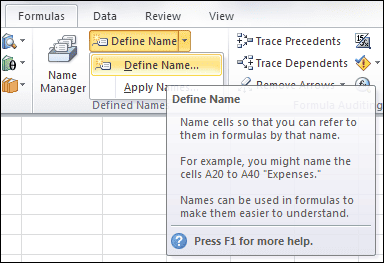# Calculate the Xth Weekday of Any Month in Excel

I’ve a simple formula for calculating the Xth Weekday of ANY month. It takes four inputs: Year, Month, Week, and Day. And requires a couple of lookup tables for data validation, one with special formatting.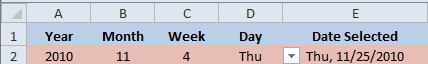### The History

It all started when my wife mentioned there were a couple of meetings she had to schedule at work, one on the second Tuesday of every month and the other on the third Tuesday of every month. That got me to thinking about how to create a formula in Excel to calculate those dates.

After getting … Read the rest

# Convert Pace to MPH and Back Again in Excel

Excel is a great tool for figuring stuff out, like for conversions that aren’t easy to do in your head. Here I’m converting Pace to MPH and then reversing the process, converting MPH to Pace, to create a conversion chart.

## My Conversion Problem for Minutes per Mile to MPH

I track my Average Pace when out walking for exercise by using the iPhone App Walkmeter, then log that information into the Lose It App. The problem I have is converting my Average Pace to Miles per Hour (MPH).

Below you can see my average … Read the rest

# Convert Seconds with the TIME Function in Excel

I often download an iTunes playlist just to see the time increments for each song, but iTunes only gives you the number of seconds for each song duration. In a previous post I spent a great deal of time showing how to use a combination of various formulas to convert these seconds, when it’s really very simple.

## Excel Formula to Convert Seconds to Minutes

You see, the Excel formula to convert seconds to minutes can be used easily with the TIME Function. All you have to do is format a worksheet cell with the … Read the rest

There are several ways to turn student scores into letter grades. I recently came across a nested IF formula that did the trick, but it seemed rather complicated.

A better solution would be to use a VLOOKUP formula with a Grade Lookup Table, but then it occurred to me that the VLOOKUP formula could stand alone by using an Array Constant.

For all these solutions I’m using the following grade scale:

• 0 – 59 = F
• 60 – 69 = D
• 70 – 79 = C
• 80 – 89 = B
• 90 – 100 =

# Nested IF Functions in Excel

The simplest Nested IF Function is using one IF Function inside another. When you have more than a few choices, nesting more IF Functions can quickly get complicated and, quite frankly, there are better ways to make decisions with Excel. Having said that, I have a simple method to account for the different choices that can arise with nested IF Functions.

## Two Conditions – Binary Outcome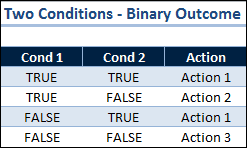The IF Function evaluates a logical test to either TRUE or FALSE. A binary outcome. One condition requires only one IF Function.

With two conditions that both … Read the rest

# The IF Function in Excel

The IF Function is used to evaluate a logical test, then if TRUE do something or if FALSE do something else. A familiar construct. The IF Function has three arguments:

IF(logical_test, [value_if_true], [value_if_false])

### Argument 1

The first argument, logical_test, is required. It’s any value or expression that can be evaluated to TRUE or FALSE. This argument can use any comparison calculation operator. There are four types:

• Arithmetic operators ( +   –   *   /   %   ^ )
• Comparison operators ( =   >   <   >=   <=   >< )
• Text concatenation operator ( & )

# Single-Cell Array Formula in Excel

A Single-cell Array formula is powerful, yet can be hard to understand. The Evaluate Formula dialog box helps by revealing array values Excel holds in internal memory.

In the spreadsheet you see pictured, a single-cell array formula for the Cumulative Plan is located in cell B2. It summarizes the historical and current day’s Plan data. The Cumulative Actual formula, in cell C2, summarizes the Actual data in the same manner.

As an introduction to Array formulas, in my last post I added two columns. The multi-cell array formulas for columns D and E are:… Read the rest

# Multi-Cell Array Formula in Excel

Arrays are difficult to understand because Excel holds them internally and it’s hard to conceptualize how operations are performed on the data. I had a vexing problem that was solved by using a single-cell array formula and wanted to share it with you.

However, because of the subject nature of Arrays, I’m going to show the solution to this problem in two parts. Here I’ll cover a Multi-Cell Array formula and my next post will talk about a Single-Cell Array formula, which, turns out, is my magic formula.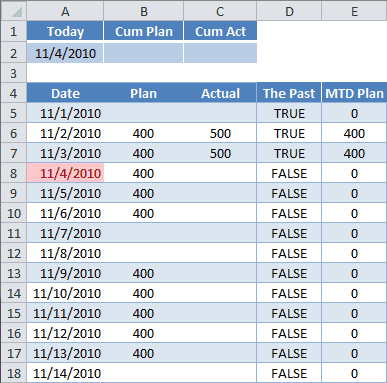### The Problem

Here’s an example worksheet. … Read the rest

# Use the DATE Function to Find the Last Day of a Month

Zero has a special meaning in the Excel date system and can be used in the DATE Function to return the last day of the month. The simplest way to show this is to use a formula to find the last day of February in the coming years.

## How to Find in Excel the Last Day of Month

If you want to find in Excel the last day of month with cell A2 containing the current year 2010, the following formula is entered into cell B2:

=DATE(A2,3,0)

and returns the result 2/28/2010.

There are three … Read the rest

# A Sparkline Example in Excel 2010

Each week I download the over-under calories report from Lose It! and dump the data into a spreadsheet. I just created my first Sparkline graphic to show the last 7 days of this data.

For this example I’ll use an OFFSET Function inside a named range, which was created in my last couple of posts.

### A Little Prep Work for a Dynamic Range

In this post I’ll use the formula:

=OFFSET(Sheet1!\$B\$1,COUNTA(Sheet1!\$B:\$B)-7,0,7)

inside the named range: LastSeven, which will … Read the rest

# Put an OFFSET Formula Inside a Named Range

In the last post I used the OFFSET Function inside the AVERAGE Function to return the last 7 days of a range. Here I’ll create a Named Range using a formula with the OFFSET Function.

I use a worksheet to inform me of the Last 7 Day Average for Over-Under Calories data, as shown below.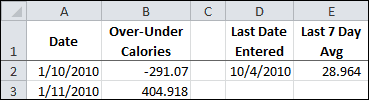Cell E2 has the following formula.

=AVERAGE(OFFSET(B1,COUNTA(B:B)-7,0,7)

The AVERAGE Function is merely taking the range reference provided by the OFFSET function to return an average.

## Create a Named Range with the OFFSET Function

I want to take everything inside the … Read the rest

# The OFFSET Function – Last 7 Data Points

In this example I’m going to use the OFFSET Function to return a range reference to the last 7 cells in a column. Of course, it will be inside the AVERAGE Function so I can see an average for the last week.

Sound complicated? Not really, it’s just an extension of something I did on a previous post for the OFFSET Function.

The example data is a spreadsheet I use to log my over-under calories to goal each day. This data is downloaded periodically and currently the data goes to row 269, as you … Read the rest

# Become the OFFSET Function and Tell a Short Story

The OFFSET Function is a difficult one to learn. I’ve decided to become the OFFSET function and tell a simple story of how I give you a value for the last cell in a range by using an example.

The example data is a spreadsheet I use to log my over-under calories to goal each day. This data is downloaded periodically and currently the data goes down to row 269, as you can see below.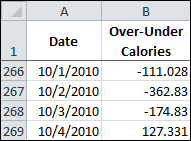I use the OFFSET Function at the top of the worksheet to inform me of the Last Date Entered in … Read the rest

# Enter a Constant Value in a Name

Once upon a time I was working with an unfamiliar Excel spreadsheet and came across a formula that used a Name, which I figured for a constant value and thought it hidden somewhere in the worksheet, yet couldn’t find it anywhere.

It never dawned on me that a value doesn’t have to reside in a worksheet cell.

## Enter a Constant Value in a Name — Excel 2007, 2010

You can put a constant value into a Name, which Excel will hold internally.

Go to Formulas, and select Define Name (Excel 2010, 2007)